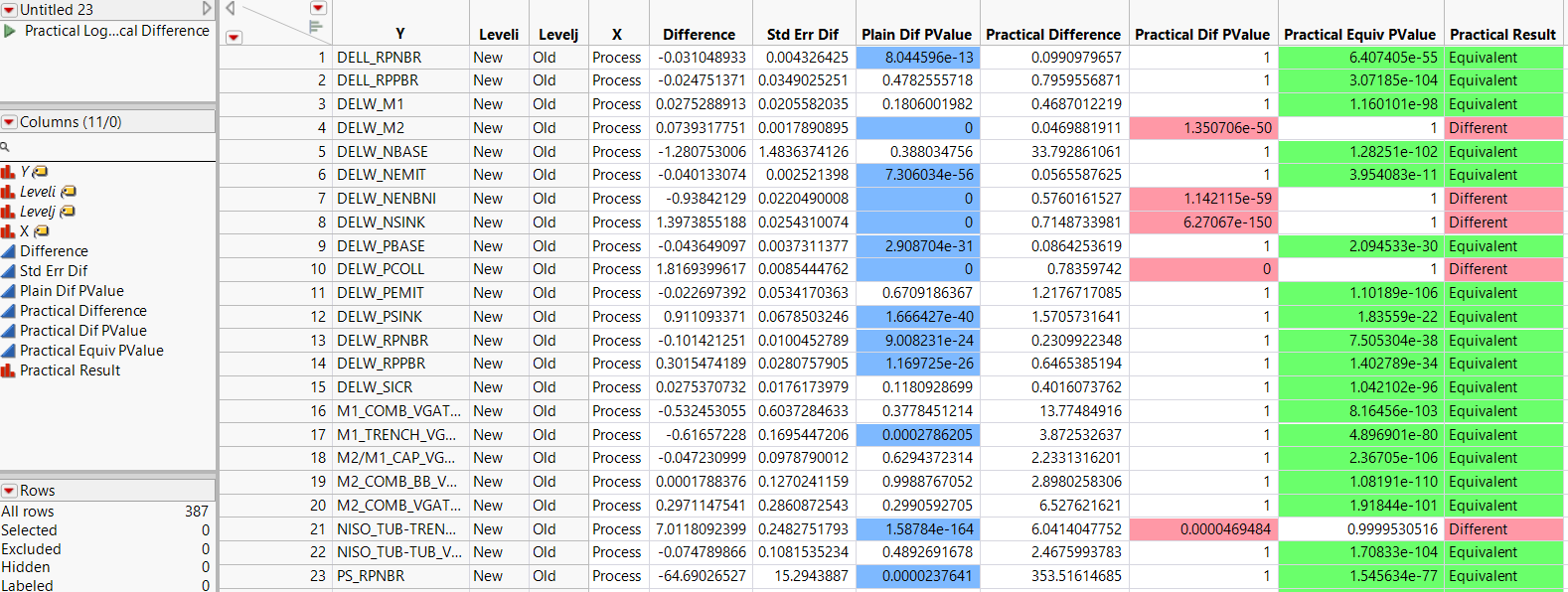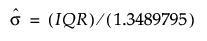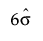Publication date: 11/10/2021

## Compare Means Data Table

When your data table consists of a large number of rows (large n), the standard error used in testing can be very small. As a result, tests might be statistically significant, when in fact, the observed difference is too small to be of practical consequence. Tests of practical significance enable you to specify the size of the difference that you consider worth detecting. This difference is called the practical difference. Instead of testing that the difference is zero, you test whether the difference exceeds the practical difference. As a result, the tests are more meaningful, and fewer tests need to be scrutinized.

Equivalence tests enable you to determine whether two levels have essentially the same effect, from a practical perspective, on the response. In other words, an equivalence test tests whether the difference is smaller than the practical difference.

The Compare Means data table provides results for both tests of practical difference and tests of practical equivalence. Each row compares a response across two levels of a categorical factor. Results of the pairwise comparisons are color-coded to facilitate interpretation. See Practical Difference for a description of how the practical difference is specified. See Example of Tests of Practical Significance and Equivalence for an example.

Figure 22.7 Compare Means Data TableThe Compare Means data table contains a script that plots Practical LogWorth by Relative Practical Difference. Relative Practical Difference is defined as the actual difference divided by the practical difference.

Y

The continuous response variables.

X

The categorical variables.

Leveli

The level of the categorical X variable.

Levelj

The level of the categorical X variable being compared to Leveli.

Difference

The estimated difference in means across the two levels. If the Robust option is selected, robust estimates of the means are used.

Std Err Diff

The standard error of the difference in means. This is a robust estimate if the Robust option is selected.

Plain Dif PValue

The p-value for the usual Student's t test for a pairwise comparison. This is the robust version of the t test when the Robust option is selected. Tests that are significant at the 0.05 level are highlighted.

Practical Difference

The difference in means that is considered to be of practical interest. If you assign a Spec Limit property to the Y variable, the practical difference is computed as the difference between the specification limits multiplied by the Practical Difference Proportion. If no Practical Difference Proportion has been specified, the Practical Difference is the difference between the specification limits multiplied by 0.10.

If you do not assign a Spec Limit property to the Y variable, an estimate of its standard deviation is computed from its interquartile range (IQR). This estimate is. The Practical Difference is computed asmultiplied by the Practical Difference Proportion. If no Practical Difference Proportion has been specified, the Practical Difference is computed asmultiplied by 0.10.

Practical Dif PValue

The p-value for a test of whether the absolute value of the mean difference in Y between Leveli and Levelj is less than or equal to the Practical Difference. A small p-value indicates that the absolute difference exceeds the Practical Difference. This indicates that Leveli and Levelj account for a difference that is of practical consequence.

Practical Equiv PValue

Uses the Two One-Sided Tests (TOST) method to test for a practical difference between the means (Schuirmann 1987). The Practical Difference specifies a threshold difference for which smaller differences are considered practically equivalent. One-sided t tests are constructed for two null hypotheses: the true difference exceeds the Practical Difference; the true difference is less than the negative of the Practical Difference. If both tests reject, this indicates that the absolute difference in the means falls within the Practical Difference. Therefore, the groups are considered practically equivalent.

The Practical Equivalence PValue is the largest p-value obtained on the one-sided t tests. A small Practical Equiv PValue indicates that the mean response for Leveli is equivalent, in a practical sense, to the mean for Levelj.

Practical Result

A description of the results of the tests for practical difference and equivalence. Values are color-coded to help identify significant results.

Different (Pink): Indicates that the absolute difference is significantly greater than the practical difference.

Equivalent (Green): Indicates that the absolute difference is significantly within the practical difference.

Inconclusive (Gray): Indicates that neither the test for practical difference nor the test for practical equivalence is significant.